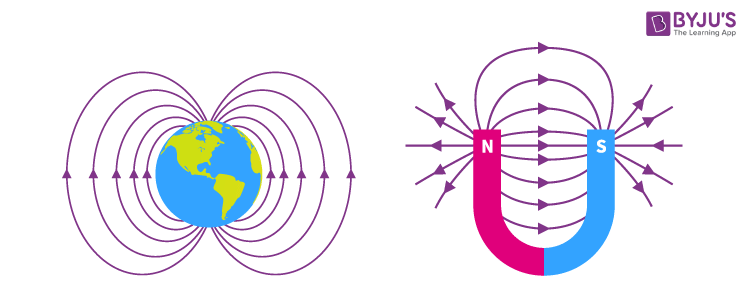# Electromagnetic Field

Welcome to class!

In today’s class, we will be talking about the electromagnetic field. Enjoy the class!

### Electromagnetic FieldAn electromagnetic field is a field representing the joint interaction of electric and magnetic forces. The electromagnetic field exerts forces on charged particles. The force on a charge q moving with a velocity v (less than the velocity of light) is given as:

F = q (E + v x B)

#### Interaction between the magnetic field and current

Force on a current-carrying conductor in a magnetic field:

A conductor carrying an electric current, when placed in a magnetic field experiences a mechanical force.

Two metal rails are fixed on each other side of a powerful horseshoe magnet. A copper rod is placed across the rails. When we pass current through this copper rod, it is observed that the copper rod rolls along the rails, towards the right. If by adjusting the rheostat, we cause more current to flow through the rod, we will observe that the rod moves faster. Thus the force on the rod increases when the current increases.

If we reverse the direction of the current flow by reversing the connections at the battery terminals, the rod will be observed to move towards the left, opposite to the previous direction of motion.

If we turn the magnet such that the magnetic field is parallel to the length of the rod.

It will be observed that the current-carrying rod remains stationary no matter the amount of current passed through it. There is, therefore, no force on the rod.

#### The force between conductors carrying current

If two parallel current-carrying conductors are arranged side by side, it is clear that each conductor is in the magnetic field of the other. We have shown that a conducting wire in a magnetic field is subject to a force. Hence each of the two conductors must exert a force on the two-conductor would be lying in the magnetic field of the other. The direction of current flow in each wire determines whether the force acting between the conductors would be attractive or repulsive.If the currents flow in the same direction. The pattern of the magnetic field which can be depicted using iron fillings and compass needle is as shown in the diagram. The force between two such conductors to move towards each other. If the current flows in opposite direction in the two conductors, the field pattern is as shown in the figure above and the force between the conductors is a repulsive force.

##### Direction of force

The direction of the force on a current-carrying conductor placed perpendicular to the magnetic field is given by Fleming’s left-hand rule which stated as follows:

##### Fleming’s left-hand rule:

It states that if the thumb, forefinger and the middle finger of the left hand are held mutually at right angles with the forefinger pointing in the direction of the magnetic field and the middle finger in the direction of the current, the thumb will point in the direction of the force.

Using this rule, the direction of the force on the conductor figure below##### Applications of the electromagnetic field
• ###### The electric motor:

The electric motor is a device for converting electrical energy into mechanical energy. It consists of (i) a rectangular coil ABCD of insulated wire, known as the armature which can turn about a fixed axis. (ii) A powerful magnetic field in which the armature turns is provided by two curved pole pieces of a powerful magnet (iii) A commutator consisting of a split copper ring, two halves of which are insulated from each half of the split copper ring (iv) Two carbon brushes B1, B2 which are made to press lightly against either side of the split-ring commutator.

(ii) Moving Coil Galvanometer

This galvanometer is one of the most sensitive and accurate methods for detecting or measuring extremely small currents or potential differences.

###### General evaluation
1. Define Electromagnetic Field

Assignment

1. Explain Fleming’s left-hand rule method.

In our next class, we will be talking about Electromagnetic Induction. We hope you enjoyed the class.

Should you have any further question, feel free to ask in the comment section below and trust us to respond as soon as possible.

How Can We Make ClassNotesNG Better - CLICK to Tell Us💃